Courses

# Differential Equations - 17

## 20 Questions MCQ Test Topic-wise Tests & Solved Examples for IIT JAM Mathematics | Differential Equations - 17

Description
This mock test of Differential Equations - 17 for Mathematics helps you for every Mathematics entrance exam. This contains 20 Multiple Choice Questions for Mathematics Differential Equations - 17 (mcq) to study with solutions a complete question bank. The solved questions answers in this Differential Equations - 17 quiz give you a good mix of easy questions and tough questions. Mathematics students definitely take this Differential Equations - 17 exercise for a better result in the exam. You can find other Differential Equations - 17 extra questions, long questions & short questions for Mathematics on EduRev as well by searching above.
QUESTION: 1

Solution:
QUESTION: 2

Solution:
QUESTION: 3

### The integrating factor of the differential equation (x3 + y2 + 2x)dx + 2ydy = 0 is

Solution:

Hint : Here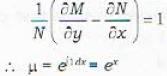QUESTION: 4

The integrating factor of the differential equation (y4 + 2y)dx + (xy3 + 2y4 - 4x)dy = 0 is

Note: A differential equation is said to be with separated variables if it can be written in the form of P(x)dx + Q(y)dy = 0

Solution:

Hint :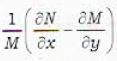is a function of y alone.

QUESTION: 5

Which of the following differential equations is with separated variables?

Solution:

Differential equation in (a) can be written as exeydx + sin x cos y dy = 0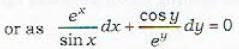QUESTION: 6

Which of the following differential equations is with separated variables?

Solution:

All the three differential equations in statements (a), (b) and (e) are with separated variables.
Do it yourself.

QUESTION: 7

The differential equation (x + y) [dx - dy] = dx + dy which is not with separated variables, can be transformed into one which is with separated variables, by the substitution

Solution:

Proof: Let x + y mv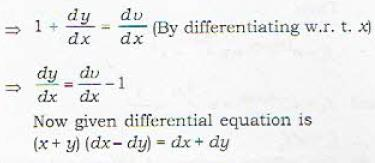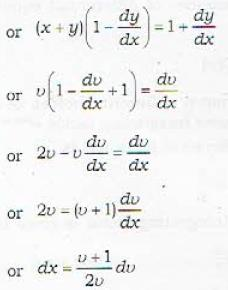QUESTION: 8

The differential equation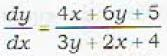which is not with separated variables, can be transformed into one which is with separated variables, by the substitution.

Solution:

Proof: Under the transformation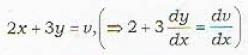the given differential equation reduces to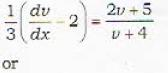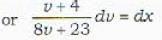Hence the variables are separated.

QUESTION: 9

Which of the following differential equations is not homogeneous one?

Solution:

Definition : Homogeneous Differential Equation.
The differential equations which can be expressed as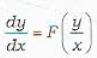are called homogeneous differential equations. Thus differential equation (a) is homogeneous because this can be written as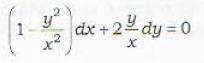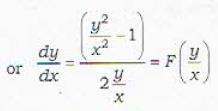Differential equation (b) is homogeneous because this can be written as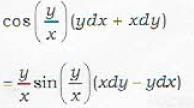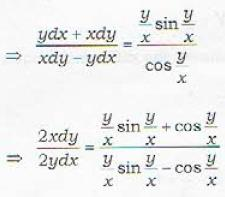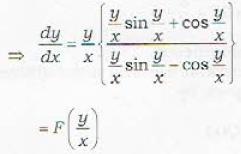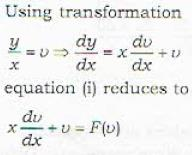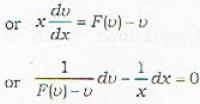which is of the type
P(u) dv - Q(x) dx = 0    ...(ii)
(i.e.seperated variables)
Hence it is exact.

QUESTION: 10

Which one of the following statements is correct?

Solution:
QUESTION: 11

The solution curves of the given differential equation xdx + ydy = 0 are given by a family of

Solution:
QUESTION: 12

The solution eurves of the given differential equation xdx - dy = 0 are given by a family of

Solution:
QUESTION: 13

A first order first degree homogeneous differential equation

Solution:
QUESTION: 14

A first order first degree homogeneous differential equation

Solution:
QUESTION: 15

Which of the following transformations reduces a homogeneous differential equation of first order and first degree into one with separated variables?

Solution:
QUESTION: 16

The transformation y = vx reduces the given homogeneous differential equation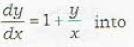Solution:

The tranformation is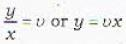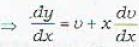∴ given differential equation reduces to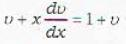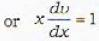QUESTION: 17

Which of the following transformations reduces the given differential equation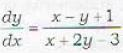in to homogeneous one?

Solution:

The transformation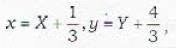given in (b) reduces the differential equation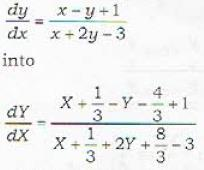which is homogeneous.

QUESTION: 18

A general linear differential equation of first order can be written as

Solution:

The most general linear first order differential equation is given by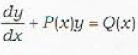Remark : DE in (a) may not be linear and DE in (c) is not most general.

QUESTION: 19

Which of the following differential equations is linear, homogeneous and of first order?

Solution:

DE in (a) is linear, homogeneous and first order.
DE in (b) is linear, first order but non-homogeneous.
DE in (c) is of first order, non-linear & non-homogeneous DE in (d) is of first order, linear but non-homogeneous.

QUESTION: 20

The integrating factor of the differential equation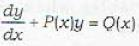depends upon

Solution:

The integrating factor is given by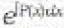Therefore the integrating factor depends upon P(x) only.